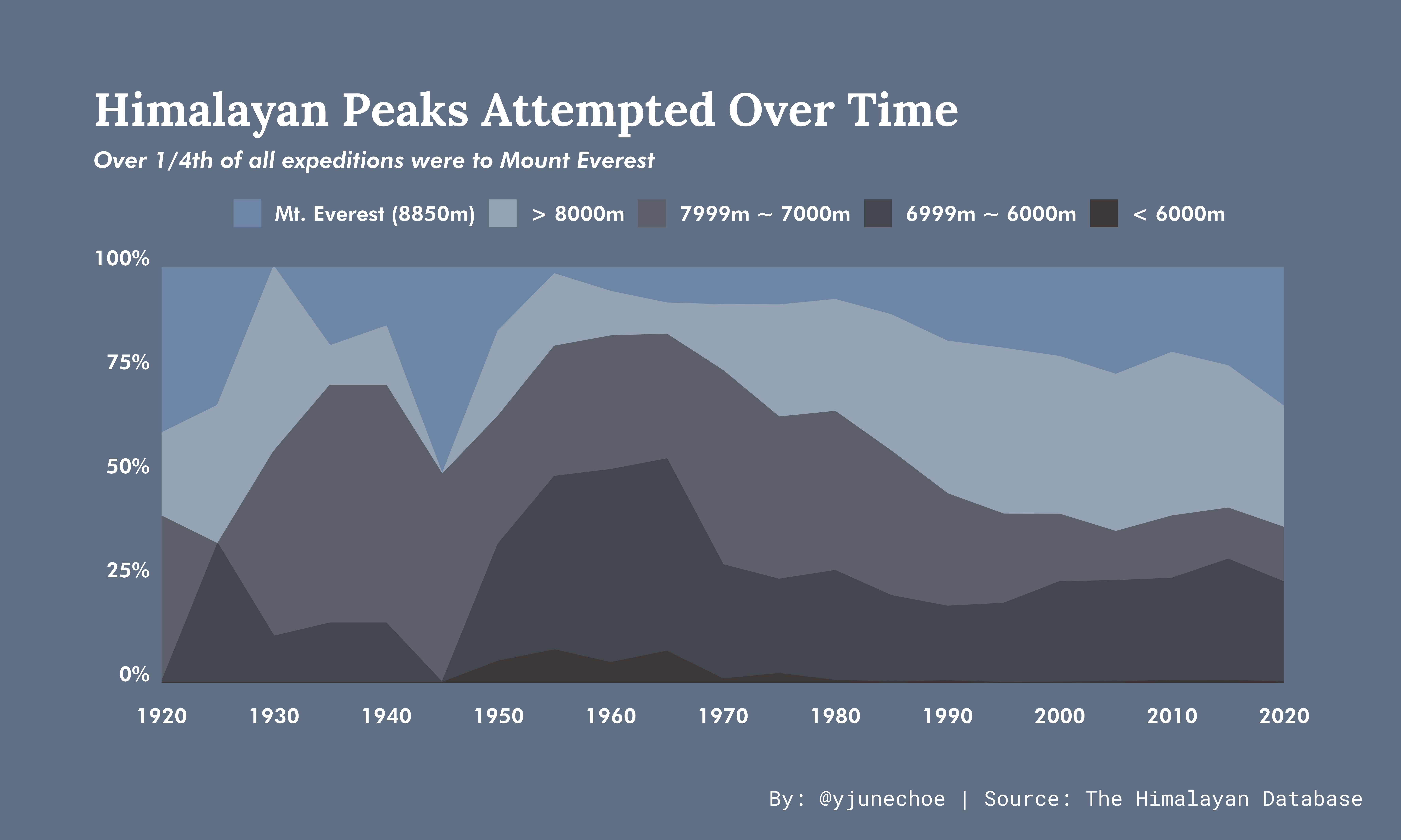# TidyTuesday 2020 week 39

Stacked area plot of the heights of Himalayan peaks attempted over the last century

June Choe (University of Pennsylvania Linguistics)https://live-sas-www-ling.pantheon.sas.upenn.edu/
09-23-2020

## Visualization### Things I learned

• Having a nice background color for the plot (and generally just working with color)

• Margin options of various kinds in `theme()`

• Using `{scales}`, `pretty_breaks()` in particular

• Using `{ragg}` to draw and save high quality plots

### Things to improve

• The subtitle is kinda boring (and the entire plot is a bit underwhelming)

• Figure out how to increase spacing between y-axis text and the plot (`hjust` is relative to each label, so doesn’t work)

## Code

Also available on github

``````library(tidyverse)

# DATA

climb_data <- tuesdata\$expeditions %>%
left_join(tuesdata\$peaks, by = "peak_name") %>%
select(peak = peak_name, year, height = height_metres) %>%
arrange(-height) %>%
mutate(height_group = fct_inorder(case_when(peak == "Everest" ~ "Mt. Everest (8850m)",
between(height, 8000, 8849) ~ "> 8000m",
between(height, 7000, 7999) ~ "7999m ~ 7000m",
between(height, 6000, 6999) ~ "6999m ~ 6000m",
TRUE ~ "< 6000m"))
) %>%
count(five_years = round(year/5) * 5, height_group) %>%
filter(five_years >= 1920) %>%
complete(five_years, height_group, fill = list(n = 0)) %>%
group_by(five_years) %>%
mutate(prop = n / sum(n)) %>%
ungroup()

# PLOT

mountain_palette <- c("#6E86A6", "#95A2B3", "#5C606A", "#44464E", "#3D3737")

climb_plot <- climb_data %>%
ggplot(aes(five_years, prop)) +
geom_area(aes(fill = height_group, color = height_group))  +
scale_fill_manual(values = mountain_palette) +
scale_color_manual(values = mountain_palette) +
coord_cartesian(xlim = c(1920, 2020), expand = FALSE) +
scale_x_continuous(breaks = scales::pretty_breaks(11)) +
scale_y_continuous(labels = scales::percent) +
labs(
title = "Himalayan Peaks Attempted Over Time",
subtitle = "Over 1/4th of all expeditions were to Mount Everest",
x = NULL, y = NULL, fill = NULL, color = NULL,
caption = "By: @yjunechoe | Source: The Himalayan Database"
) +
theme_classic(base_family = "Futura Hv BT", base_size = 16) +
theme(
plot.title.position = "plot",
plot.title = element_text(size = 28, color = "white", family = "Lora", face = "bold"),
plot.subtitle = element_text(size = 14, color = "white", face = "italic"),
plot.margin = margin(2, 2.5, 2, 2, 'cm'),
plot.caption = element_text(color = "white", family = "Roboto Mono", hjust = 1.15, vjust = -13),
legend.position = "top",
legend.direction = "horizontal",
legend.text = element_text(color = "white"),
legend.background = element_rect(fill = NA),
axis.text = element_text(color = "white"),
axis.text.y = element_text(vjust = -.1),
axis.text.x = element_text(vjust = -2),
axis.ticks = element_blank(),
axis.line = element_blank(),
panel.background = element_blank(),
plot.background = element_rect(fill = "#606F84", color = NA)
)

# SAVE

pngfile <- fs::path(getwd(), "plot.png")
ragg::agg_png(
pngfile,
width = 60,
height = 36,
units = "cm",
res = 300,
scaling = 2
)
plot(climb_plot); invisible(dev.off())
``````